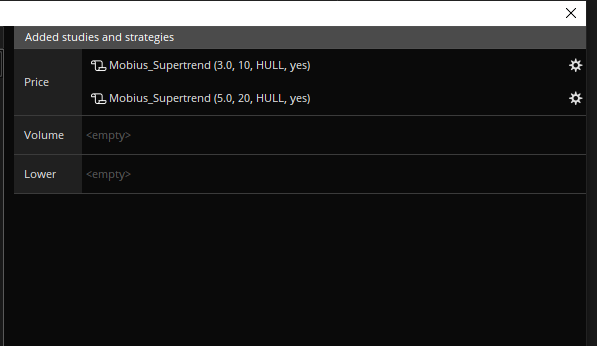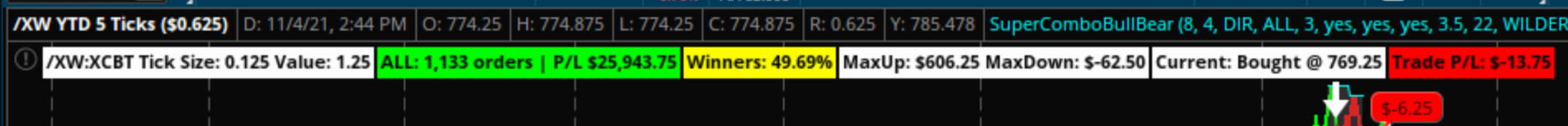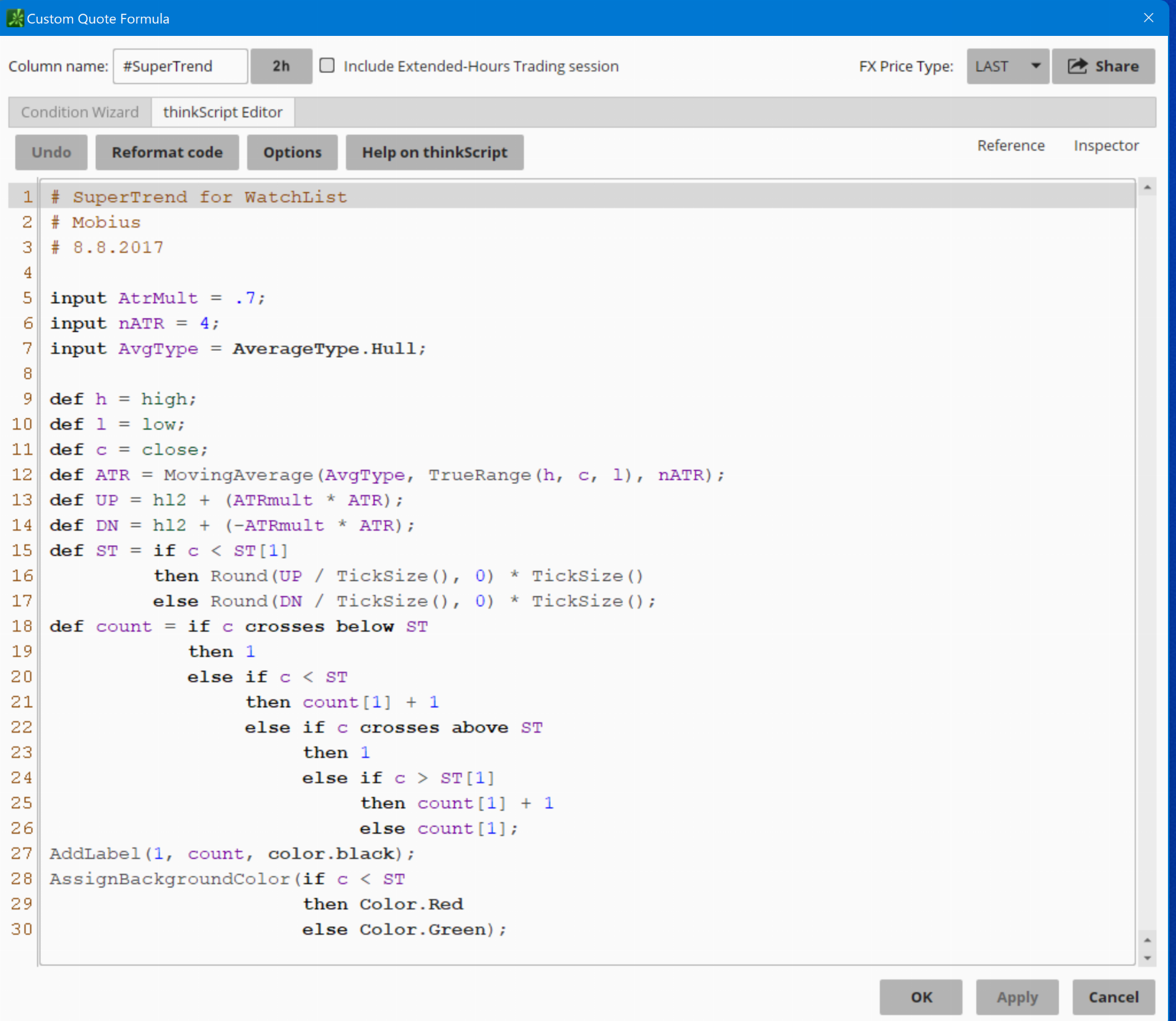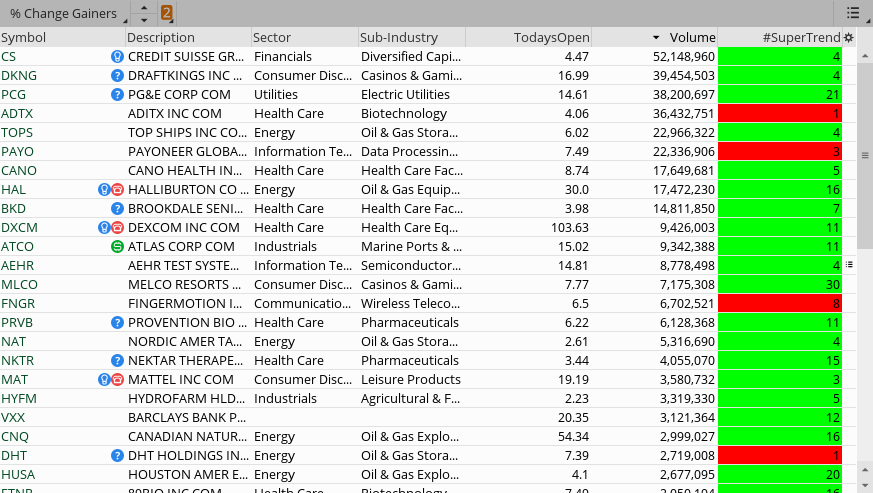# Supertrend Indicator by Mobius for ThinkorSwim

@kls06541
Apply the indicator to the upper chart twice and use the settings in my previous post.Cool and the indicator is not recommended for below 5min chart what time chart do you have this on?

How would I add a ding sound (Sound.DING) when either the "Sell @" or "Buy @" chart bubbles are added?

AddChartBubble(close[n] crosses below ST[n], low[n+1] + TickSize() * n, "Sell @ " + low[n1], color.Cyan, yes); AddChartBubble(close[n] crosses above ST[n], high[n+1] - TickSize() * n, "Buy @ " + high[n1], color.Yellow, no);

Thank you,

How would I add a ding sound (Sound.DING) when either the "Sell @" or "Buy @" chart bubbles are added?

AddChartBubble(close[n] crosses below ST[n], low[n+1] + TickSize() * n, "Sell @ " + low[n1], color.Cyan, yes); AddChartBubble(close[n] crosses above ST[n], high[n+1] - TickSize() * n, "Buy @ " + high[n1], color.Yellow, no);

Thank you,

This should help

Code:
``````input alerts = yes;

Is it possible to change the chart bubbles to arrows instead?

Is it possible to change the chart bubbles to arrows instead?
Would like to see this too

You can try this template I use for my studies, pieced it together and modified it from here and there, most from linus' SuperCombo. Basically, just plug in what triggers your buy and sell signals (and stops if you use them) and it will put a bar on top with your overall p/l for the given timeframe. This isn't a strategy so a report isn't given, but it does give a quick p/l so you can change settings and instantly see the effect. Note, this won't account for any fees or commissions, so if doing futures, have to take off the fees per order.Code:
``````input showSignals = yes;
input showLabels  = yes;
input showBubbles = yes;
input useStops = no;

############################################
##  Create Signals - FILL IN THIS SECTION
############################################

def BuySignal  ; # insert condition to create long position
def SellSignal ; # insert condition to create short position
def BuyStop  = if !useStops then 0 else 0  ; # insert condition to stop in place of the 0 after else
def SellStop = if !useStops then 0 else 0  ; # insert condition to stop in place of the 0 after else

#######################################
##  Maintain the position of trades
#######################################

def CurrentPosition;  # holds whether flat = 0 long = 1 short = -1

if (BarNumber()==1) OR isNaN(CurrentPosition) {
CurrentPosition = 0;
}else{
if CurrentPosition == 0 {            # FLAT
CurrentPosition = 1;
} else if (SellSignal){
CurrentPosition = -1;
} else {
CurrentPosition = CurrentPosition;
}
} else if CurrentPosition == 1 {      # LONG
if (SellSignal){
CurrentPosition = -1;
CurrentPosition = 0;
} else {
CurrentPosition = CurrentPosition;
}
} else if CurrentPosition == -1 {     # SHORT
CurrentPosition = 1;
} else if (SellStop){
CurrentPosition = 0;
} else {
CurrentPosition = CurrentPosition;
}
} else {
CurrentPosition = CurrentPosition;
}
}

def isLong  = if CurrentPosition == 1 then 1 else 0;
def isShort = if CurrentPosition == -1 then 1 else 0;
def isFlat  = if CurrentPosition == 0 then 1 else 0;

#######################################
##  Plot the Signals
#######################################

Plot BuySig = if (!isLong and BuySignal and showSignals) then 1 else 0;

Plot SellSig = if (!isShort and SellSignal and showSignals) then 1 else 0;
SellSig.AssignValueColor(color.white);
SellSig.SetPaintingStrategy(PaintingStrategy.BOOLEAN_ARROW_DOWN);
SellSig.SetLineWeight(3);

Plot BuyStpSig = if (BuyStop and isLong and showSignals) then 1 else 0;

Plot SellStpSig = if (SellStop and isShort and showSignals) then 1 else 0;
SellStpSig.AssignValueColor(color.gray);
SellStpSig.SetPaintingStrategy(PaintingStrategy.BOOLEAN_ARROW_UP);
SellStpSig.SetLineWeight(3);

#######################################
##  Orders
#######################################

def isOrder = if CurrentPosition == CurrentPosition then 0 else 1; # Status changed so it's a new order
def orderPrice = if (isOrder and (BuySignal or SellSignal)) then open[-1] else orderPrice;

#######################################
##  Price and Profit
#######################################

def profitLoss;

if (!isOrder){
profitLoss = 0;
} else if ((isOrder and isLong) and (SellSig or BuyStpSig)){
profitLoss = close - orderPrice;
} else if ((isOrder and isShort) and (BuySig or SellStpSig)) {
profitLoss = orderPrice - close;
} else {
profitLoss = 0;
}

def profitLossSum = compoundValue(1, if isNaN(isOrder) then 0 else if isOrder then profitLossSum + profitLoss else profitLossSum, 0);
def profitWinners = compoundValue(1, if isNaN(isOrder) then 0 else if isOrder and profitLoss > 0 then profitWinners + 1 else profitWinners, 0);
def profitLosers = compoundValue(1, if isNaN(isOrder) then 0 else if isOrder and profitLoss < 0 then profitLosers + 1 else profitLosers, 0);
def profitPush = compoundValue(1, if isNaN(isOrder) then 0 else if isOrder and profitLoss == 0 then profitPush + 1 else profitPush, 0);
def TradePL = If isLong then Round(((close - orderprice)/TickSize())*TickValue()) else if isShort then Round(((orderPrice - close)/TickSize())*TickValue()) else 0; # current trade p/l
def dollarProfitLoss = round((profitLoss/Ticksize())*Tickvalue()); # per trade for chart bubbles
def biggestWin = compoundValue(1, if isNaN(isOrder) then 0 else if isOrder and (dollarProfitLoss > 0) and (dollarProfitLoss > biggestWin) then dollarProfitLoss else biggestWin, 0);
def biggestLoss = compoundValue(1, if isNaN(isOrder) then 0 else if isOrder and (dollarProfitLoss < 0) and (dollarProfitLoss < biggestLoss) then dollarProfitLoss else biggestLoss, 0);

def orderCount = (profitWinners+profitLosers+profitPush);
def PCTWin = round((profitWinners/orderCount)*100,2);

#######################################
##  Create Labels
#######################################

AddLabel(yes, GetSymbol()+" Tick Size: "+TickSize()+" Value: "+TickValue(), color.white);
AddLabel(showSignals and showLabels, "Orders: " + orderCount + " P/L: " + AsDollars(profitLossSum), if profitLossSum > 0 then Color.GREEN else if profitLossSum < 0 then Color.RED else Color.GRAY);
AddLabel(yes, "Winners: "+ PCTWin +"%",if PCTWin > 50 then color.green else if PCTWin > 40 then color.yellow else color.gray);
AddLabel(yes, "MaxUp: "+ AsDollars(biggestWin) +" MaxDown: "+AsDollars(biggestLoss), color.white);
AddLabel(if !IsNan(CurrentPosition) then 1 else 0, "Current: "+ (If isLong then "Bought" else "Sold") + " @ "+orderPrice, color.white);

#######################################
##  Chart Bubbles for Profit/Loss
#######################################

AddChartBubble(showSignals and showBubbles and isOrder and isLong, low, "\$"+dollarProfitLoss, if dollarProfitLoss == 0 then Color.LIGHT_GRAY else if dollarProfitLoss > 0 then Color.GREEN else color.Red, 1);
AddChartBubble(showSignals and showBubbles and isOrder and isShort, high,  "\$"+dollarProfitLoss, if dollarProfitLoss == 0 then Color.LIGHT_GRAY else if dollarProfitLoss > 0 then Color.GREEN else color.Red, 0);``````
the above code produce the following error:

Value never assigned to BuySignal at 13:5
Value never assigned to SellSignal at 20:5

the above code produce the following error:

Value never assigned to BuySignal at 13:5
Value never assigned to SellSignal at 20:5
The directions state:
1. You can try this template
From the error codes that you generated, it would appear that whatever you plugged in as your triggers for your buy and sell signals was not valid.
Without seeing how you coded your plugs, it is not possible to provide any further assistance.

Last edited:
can anyone make the ST lines like trailing stop, for example, only allow the ST line to go up in bullish trend and only allowed to go down in bearish ones

Last edited by a moderator:
Last edited by a moderator:
•MLlalala
Hi I'm trying to apply a condition for the scanner where the supertrend Up line is above the 200sma but I can't figure it out for the life of me.
So what I've done in the past is use a simple script in custom study such as: SimpleMovingAvg(length = 100) is greater than SimpleMovingAvg(length = 200)from 1 bars ago
I tried using: Supertrend(1) is greater than SimpleMovingAvg(length = 200)from 1 bars ago but it didn't work.
I think I need to add a few line of codes into the original Mobius supertrend scanner script itself but have no clue what to add, does anyone have any ideas?

I have this supertrend indicator long time ago and it worked very well for me, I got it from here and I think it was posted by Mobius. It has the pivots, resistance and support lines and it can work for any time frame.

#Public Indicator
#Special Thanks to Mobius who created original super trend indicator. This is a spin off of his work. All credits go to him. This software is free.
#CREATED 09/12/2020

input AtrMult = 0.7;
input nATR = 4;
input AvgType = AverageType.HULL;
input PaintBars = yes;
def ATR = MovingAverage(AvgType, TrueRange(high, close, low), nATR);
def UP = HL2 + (AtrMult * ATR);
def DN = HL2 + (-AtrMult * ATR);
def ST = if close < ST then UP else DN;
plot SuperTrend = ST;

SuperTrend.AssignValueColor(if close < ST then Color.GREEN else color.RED);

AssignPriceColor(if PaintBars and close < ST

then Color.RED

else if PaintBars and close > ST

then Color.GREEN

else Color.CURRENT);

#AddChartBubble(close crosses below ST, low, low, #color.Dark_Gray);
#AddChartBubble(close crosses above ST, high, high, #color.Dark_Gray, no);
# End Code SuperTrend# Mobius
# SuperTrend
# Chat Room Request

plot SuperTrendUp = close crosses above ST;
plot SuperTrendDown = close crosses below ST;
SuperTrend.AssignValueColor(if close < ST then Color.RED else Color.GREEN);
SuperTrendUp.SetDefaultColor(Color.YELLOW);
SuperTrendUp.setPaintingStrategy(PaintingStrategy.BOOLEAN_ARROW_UP);
SuperTrendDown.SetDefaultColor(Color.PINK);
SuperTrendDown.setPaintingStrategy(PaintingStrategy.BOOLEAN_ARROW_DOWN);

def bullish = close crosses below ST;
def bearish = close crosses above ST;

#ProjectionPivots_v03_JQ
#03.04.2019
#Original Code and Concept by Mobius:
# V01.08.2012 Projection Pivots
# mobius

# Notes:
# 03.04.2019 added linits on extensions
# 03.05.2019 adjusted limits on extensions by adding user input upper and lower extenion percent limits

#declare Once_Per_Bar;
#Inputs
input n = 21;
input showLines = yes;
input showValues = no;
input showBarNumbers = no;
input ExtensionLengthBars = 20; # added to control length of Entension
input UpperExtensionPercentLimit = 5;
input LowerExtensionPercentLimit = 5;
input DisplayLabel = yes; #JQ 7.8.2018 added
addlabel (DisplayLabel, "Projection Pivots n:" + n + " " , color.WHITE); #JQ 7.8.2018 added

# code from various sources including Mobius, NoLongerNube and others
# Comment out unnecessary portions to preserve tos memory and enhance speed

# Universal Definitions using Padawan variable naming convention (JQ) v03.04.2019
# iData Definitions
def vHigh = high; # creates the variable vHigh. Use of the variable reduce data calls to tos iData server
# def initHigh = CompoundValue(1, high, high); # creates and initialized variable for High
def vLow = low;
# def initLow = CompoundValue(1, low, low);
def vOpen = open;
# def initOpen = CompoundValue(1, open, open);
def vClose = close;
# def initClose = CompoundValue(1, close, close);
def vVolume = volume;
# def initVolume = CompoundValue(1, volume, volume);
def nan = Double.NaN;
# Bar Time & Date
def bn = BarNumber();
def currentBar = HighestAll(if !IsNaN(vHigh) then bn else nan);
# def Today = GetDay() ==GetLastDay();
# def time = GetTime();
# def GlobeX = GetTime() < RegularTradingStart(GetYYYYMMDD());
# def globeX_v2 = if time crosses below RegularTradingEnd(GetYYYYMMDD()) then bn else GlobeX;
# def RTH = GetTime() > RegularTradingStart(GetYYYYMMDD());
# def RTH_v2 = if time crosses above RegularTradingStart(GetYYYYMMDD()) then bn else RTH;

# bars that start and end the sessions #(borrowed from nube)
# def rthStartBar = CompoundValue(1,
# if !IsNaN(vClose)
# && time crosses above RegularTradingStart(GetYYYYMMDD())
# then bn
# else rthStartBar, 0);
# def rthEndBar = CompoundValue(1,
# if !IsNaN(vClose)
# && time crosses above RegularTradingEnd(GetYYYYMMDD())
# then bn
# else rthEndBar, 1);
# def globexStartBar = CompoundValue(1,
# if !IsNaN(vClose)
# && time crosses below RegularTradingEnd(GetYYYYMMDD())
# then bn
# else globexStartBar, 1);
# def rthSession = if bn crosses above rthStartBar #+ barsExtendedBeyondSession
# then 1
# else if bn crosses above rthEndBar #+ barsExtendedBeyondSession
# then 0
# else rthSession;

# Bubble Locations
def x_AxisLastExpansionBar = BarNumber() == HighestAll(BarNumber()); #corrected 11.12.2018 (JQ)
# syntax: addChartBubble(x_AxisLastExpansionBar, y-axis coordinate," text", Color.LIME); #verified 12.25.2018 (JQ)

def PH;
def PL;
def hh = fold i = 1 to n + 1
with p = 1
while p
do vHigh > getValue(vHigh, -i);
PH = if (bn > n and
vHigh == highest(vHigh, n) and
hh)
then vHigh
else double.NaN;
def ll = fold j = 1 to n + 1
with q = 1
while q
do vLow < getValue(low, -j);
PL = if (bn > n and
vLow == lowest(vLow, n) and
ll)
then vLow
else double.NaN;
def PHBar = if !isNaN(PH)
then bn
else PHBar;
def PLBar = if !isNaN(PL)
then bn
else PLBar;
def PHL = if !isNaN(PH)
then PH
else PHL;
def priorPHBar = if PHL != PHL
then PHBar
else priorPHBar;
def PLL = if !isNaN(PL)
then PL
else PLL;
def priorPLBar = if PLL != PLL
then PLBar
else priorPLBar;
def HighPivots = bn >= highestAll(priorPHBar);
def LowPivots = bn >= highestAll(priorPLBar);
def FirstRpoint = if HighPivots
then bn - PHBar
else 0;
def PriorRpoint = if HighPivots
then bn - PriorPHBar
else 0;
def RSlope = (getvalue(PH, FirstRpoint) - getvalue(PH, PriorRpoint))
/ (PHBar - PriorPHBar);
def FirstSpoint = if LowPivots
then bn - PLBar
else 0;
def PriorSpoint = if LowPivots
then bn - PriorPLBar
else 0;
def SSlope = (getvalue(PL, FirstSpoint) - getvalue(PL, PriorSpoint))
/ (PLBar - PriorPLBar);
def RExtend = if bn == highestall(PHBar)
then 1
else RExtend;
def SExtend = if bn == highestall(PLBar)
then 1
else SExtend;

plot pivotHigh = if HighPivots
then PH
else double.NaN;
pivotHigh.SetDefaultColor(GetColor(1));
pivotHigh.setPaintingStrategy(PaintingStrategy.VALUES_ABOVE);
pivotHigh.setHiding(!showValues);

plot pivotHighLine = if PHL > 0 and
HighPivots
then PHL
else double.NaN;
pivotHighLine.SetPaintingStrategy(PaintingStrategy.DASHES); # Mobius original was DASHES
pivotHighLine.setHiding(!showLines);

plot RLine = pivotHigh;
RLine.enableApproximation();
RLine.SetDefaultColor(Color.LIGHT_GRAY);
RLine.SetStyle(Curve.Short_DASH);

# Added code to limit resistance estension line (JQ 03.04.2019)
def calc_ResistanceExtension = if RExtend
then (bn - PHBar) * RSlope + PHL
else double.NaN;
plot line_ResistanceExtension = if bn <= (Currentbar + ExtensionLengthBars)
and calc_ResistanceExtension >= (lowestall(vLow) * (1-(lowerExtensionPercentLimit/100)))
and calc_ResistanceExtension <= (Highestall(vHigh) * (1 + (upperExtensionPercentLimit/100)))
then calc_ResistanceExtension else double.nan;
line_ResistanceExtension.SetStyle(Curve.Short_DASH);
line_ResistanceExtension.SetDefaultColor(color.LIGHT_GRAY); #was 7
line_ResistanceExtension.setLineWeight(1);

# Low Plots
plot pivotLow = if LowPivots
then PL
else double.NaN;
pivotLow.setDefaultColor(GetColor(4));
pivotLow.setPaintingStrategy(PaintingStrategy.VALUES_BELOW);
pivotLow.setHiding(!showValues);

plot pivotLowLine = if PLL > 0 and
LowPivots
then PLL
else double.NaN;
pivotLowLine.SetPaintingStrategy(PaintingStrategy.DASHES); # Mobius original was DASHES
pivotLowLine.setHiding(!showLines);

plot SupportLine = pivotLow;
SupportLine.enableApproximation();
SupportLine.SetDefaultColor(color.LIGHT_GRAY);
SUpportLine.SetStyle(Curve.Short_DASH);

# Added code to limit support estension line (JQ 03.04.2019)
def calc_SupportExtension = if SExtend
then (bn - PLBar) * SSlope + PLL
else double.NaN;
plot line_SupportExtension = if bn <= (Currentbar + ExtensionLengthBars)
and calc_SupportExtension >= (lowestall(vLow) * (1-(lowerExtensionPercentLimit/100)))
and calc_SupportExtension <= (Highestall(vHigh) * (1 + (upperExtensionPercentLimit/100)))
then calc_supportExtension else double.nan;
line_SupportExtension.SetDefaultColor(color.LIGHT_GRAY); #was 7
line_SupportExtension.SetStyle(Curve.Short_DASH);
line_SupportExtension.setLineWeight(1);

plot BarNumbersBelow = bn;
BarNumbersBelow.SetDefaultColor(GetColor(0));
BarNumbersBelow.setHiding(!showBarNumbers);
BarNumbersBelow.SetPaintingStrategy(PaintingStrategy.VALUES_BELOW);

plot PivotDot = if !isNaN(pivotHigh)
then pivotHigh
else if !isNaN(pivotLow)
then pivotLow
else double.NaN;
pivotDot.SetDefaultColor(GetColor(7));
pivotDot.SetPaintingStrategy(PaintingStrategy.POINTS);
pivotDot.SetLineWeight(3);
##

# End Code

•Topcat357 and //YYZ//
Can anybody teach me how to use this Super Trend WatchList? I thought it is for scan. But it cannot be saved because there is no plot. I cannot find a way to make a watchList based on above code.
I tried to add the script but can't figure it out... I want it to just show new signals green color for UP or BUY, and red color for DOWN or sell.. could you modified the script please? I'm going to use it for my watchlist column...

I am not showing any errors:
make sure you are using this script:#### Attachments

Last edited:
Thanks so much for this indicator. Can I change the code to remove the color lines following along the bars or change the color of these lines.?

hello everyone, a quick question, can someone here can add a buy and sell orders strategy to back test this system? just a basic buy order and sell order with the signal. thank you in advance

hello everyone, a quick question, can someone here can add a buy and sell orders strategy to back test this system? just a basic buy order and sell order with the signal. thank you in advance
meaning for the mobius super trend V3

Last edited by a moderator:

### Not the exact question you're looking for?

87k+ Posts
378 Online## The Market Trading Game Changer

Join 2,500+ subscribers inside the useThinkScript VIP Membership Club
• Exclusive indicators
• Proven strategies & setups
• Private Discord community
• Exclusive members-only content
• 1 full year of unlimited support

What is useThinkScript?

useThinkScript is the #1 community of stock market investors using indicators and other tools to power their trading strategies. Traders of all skill levels use our forums to learn about scripting and indicators, help each other, and discover new ways to gain an edge in the markets.

How do I get started?

We get it. Our forum can be intimidating, if not overwhelming. With thousands of topics, tens of thousands of posts, our community has created an incredibly deep knowledge base for stock traders. No one can ever exhaust every resource provided on our site.

If you are new, or just looking for guidance, here are some helpful links to get you started.

What are the benefits of VIP Membership?